Let's see what's really going on when we multiply two numbers.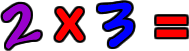This means that you have two groups of 3!Put the two groups together...  How many triangles do you have?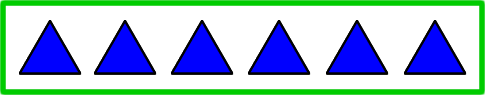Count them...  One, two, three, four, five, six! So, our answer is: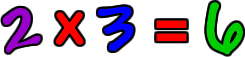Let's do another one!  This one has the numbers switched around.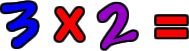This means that you have three groups of 2!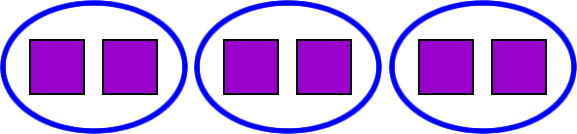Put the three groups together...  How many squares do you have?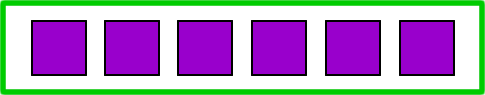Count them...  One, two, three, four, five, six! So, our answer is: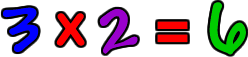Hey, that's the same answer we got with 2 x 3!  But, we put the six together a different way.  Look at them both again to see the difference!